Latest Banking jobs   »   Quantitative Aptitude Quiz For RRB PO...

# Quantitative Aptitude Quiz For RRB PO Mains 2021- 6th September

Directions (1-5): Solve the given quadratic equations and mark the correct option based on your answer—
(a) x > y
(b) x ≥ y
(c) x < y
(d) x ≤ y
(e) x = y or no relation can be established between x and y.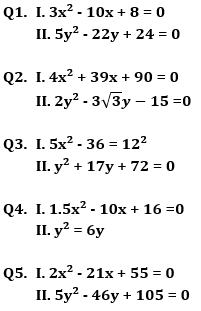Directions (6-10): In each of these questions, two equations (I) and (II) are given. You have to solve both the equations and give answer
(a) if x>y
(b) if x≥y
(c) if x<y
(d) if x ≤y
(e) if x = y or No relation can be established between x and y.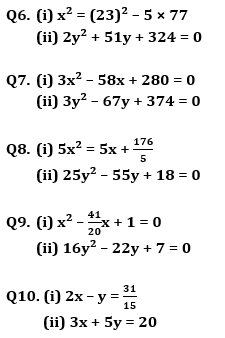Solutions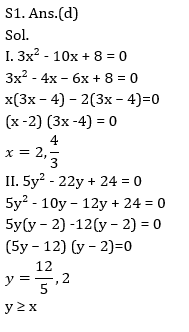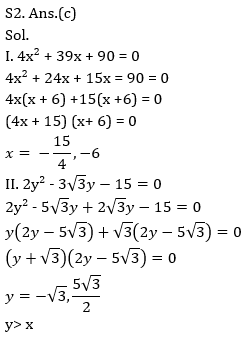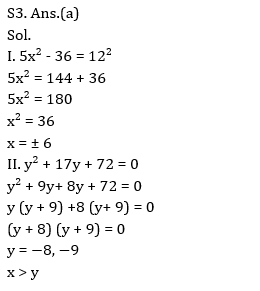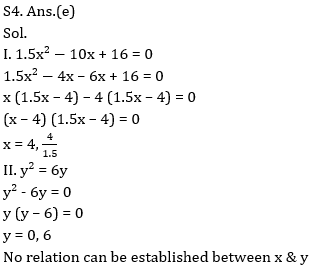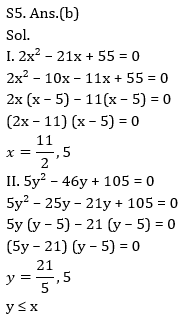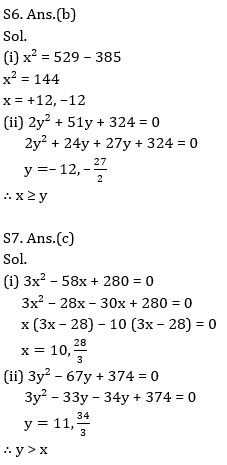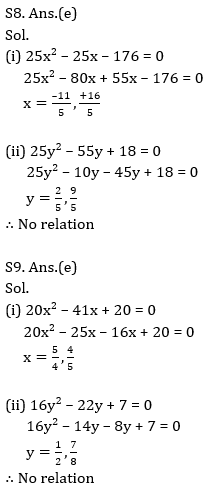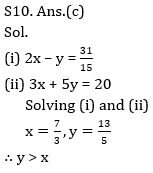Click Here to Register for Bank Exams 2021 Preparation Material#### Congratulations!Download Hindu Review of October 2021: Free PDF